## This Week's Finds in Mathematical Physics (Week 258)

#### John Baez

week258

Happy Thanksgiving! Today I'll talk about a conjecture by Deligne on Hochschild cohomology and the little 2-cubes operad.

But first I'll talk about... dust!

I began "week257" with some chat about about dust in a binary star system called the Red Rectangle. So, it was a happy coincidence when shortly thereafter, I met an expert on interstellar dust.

I was giving some talks at James Madison University in Harrisonburg, Virginia. They have a lively undergraduate physics and astronomy program, and I got a nice tour of some labs - like Brian Utter's granular physics lab.

It turns out nobody knows the equations that describe the flow of grainy materials, like sand flowing through an hourglass. It's a poorly understood state of matter! Luckily, this is a subject where experiments don't cost a million bucks.

Brian Utter has a nice apparatus consisting of two clear plastic sheets with a bunch of clear plastic disks between them - big "grains". And, he can make these grains "flow". Since they're made of a material that changes its optical properties under stress, you can see "force chains" flicker in and out of existence as lines of grains get momentarily stuck and then come unstuck!

These force chains look like bolts of lightning: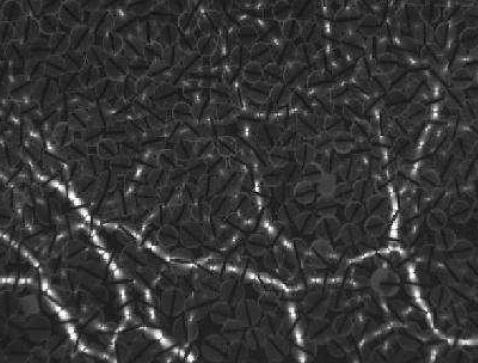1) Brian Utter and R. P. Behringer, Self-diffusion in dense granular shear flows, Physical Review E 69, 031308 (2004). Also available as arXiv:cond-mat/0402669.

I wonder if conformal field theory could help us understand these simplified 2-dimensional models of granular flow, at least near some critical point between "stuck" and "unstuck" flow. Conformal field theory tends to be good at studying critical points in 2d physics.

Anyway, I'm digressing. After looking at a chaotic double pendulum in another lab, I talked to Harold Butner about his work using radio astronomy to study interstellar dust.

He told me that the dust in the Red Rectangle contains a lot of PAHs - "polycyclic aromatic hydrocarbons". These are compounds made of hexagonal rings of carbon atoms, with some hydrogens along the edges.On Earth you can find PAHs in soot, or the tarry stuff that forms in a barbecue grill. Wherever carbon-containing materials suffer incomplete combustion, you'll find PAHs.

Benzene has a single hexagonal ring, with 6 carbons and 6 hydrogens. You've probably heard about naphthalene, which is used for mothballs. This consists of two hexagonal rings stuck together. True PAHs have more. Anthracene and phenanthrene consist of three rings:Phenanthrene

Napthacene, pyrene, triphenylene and chrysene consist of four: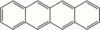Napthacene (also known as tetracene)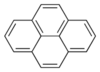Pyrene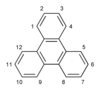Triphenylene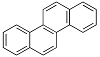Chrysene
and so on:

2) Wikipedia, Polycyclic aromatic hydrocarbon, http://en.wikipedia.org/wiki/Polycyclic_aromatic_hydrocarbon

In 2004, a team of scientists discovered anthracene and pyrene in the Red Rectangle! This was first time such complex molecules had been found in space:

3) Uma P. Vijh, Adolf N. Witt, and Karl D. Gordon, Small polycyclic aromatic hydrocarbons in the Red Rectangle, The Astrophysical Journal, 619 (2005) 368-378. Also available as arXiv:astro-ph/0410130.

By now, lots of organic molecules have been found in interstellar or circumstellar space. There's a whole "ecology" of organic chemicals out there, engaged in complex reactions. Life on planets might someday be seen as just an aspect of this larger ecology.

I've read that about 10% of the interstellar carbon is in the form of PAHs - big ones, with about 50 carbons per molecule. They're common because they're incredibly stable. They've even been found riding the shock wave of a supernova explosion!

PAHs are also found in meteorites called "carbonaceous chondrites". These space rocks contain just a little carbon - about 3% by weight. But, 80% of this carbon is in the form of PAHs.

Here's an interview with a scientist who thinks PAHs were important precursors of life on Earth:

5) Aromatic world, interview with Pascale Ehrenfreund, Astrobiology Magazine, available at http://www.astrobio.net/news/modules.php?op=modload&name=News&file=article&sid=1992

And here's a book she wrote, with a chapter on organic molecules in space:

6) Pascale Ehrenfreud, editor, Astrobiology: Future Perspectives, Springer Verlag, 2004.

Harold Butner also told me about dust disks that have been seen around the nearby stars Vega and Epsilon Eridani. By examining these disks, we may learn about planets and comets orbiting these stars. Comets emit a lot of dust, and planets affect its motion.

Mathematicians will be happy to know that symplectic geometry is required to simulate the motion of this dust:

7) A. T. Deller and S. T. Maddison, Numerical modelling of dusty debris disks, Astrophys. J. 625 (2005), 398-413. Also available as arXiv:astro-ph/0502135

Okay... now for a bit about Hochschild cohomology. I want to outline a conceptual proof of Deligne's conjecture that the cochain complex for Hochschild cohomology is an algebra for the little 2-cubes operad. There are a bunch of proofs of this by now. Here's a great introduction to the story:

8) Maxim Kontsevich, Operads and motives in deformation quantization, available as arXiv:math/9904055.

I was inspired to seek a more conceptual proof by some conversations I had with Simon Willerton in Sheffield this summer, and this paper of his:

9) Andrei Caldararu and Simon Willerton, The Mukai pairing, I: a categorical approach, available as arXiv:0707.2052.

But, while trying to write up a sketch of this more conceptual proof, I discovered that it had already been worked out:

10) Po Hu, Igor Kriz and Alexander A. Voronov, On Kontsevich's Hochschild cohomology conjecture, available at arXiv:math.AT/0309369.

This was a bit of a disappointment - but also a relief. It means I don't need to worry about the technical details: you can just look them up! Instead, I can focus on sketching the picture I had in mind.

If you don't know anything about Hochschild cohomology, don't worry! It only comes in at the very end. In fact, the conjecture follows from something simpler and more general. So, what you really need is a high tolerance for category theory, homological algebra and operads.

First, suppose we have any monoidal category. Such a category has a tensor product and a unit object, which we'll call I. Let end(I) be the set of all endomorphisms of this unit object.

Given two such endomorphisms, say

f: I → I

and

g: I → I

we can compose them, getting

f o g: I → I

This makes end(I) into a monoid. But we can also tensor f and g, and since I ⊗ I is isomorphic to I in a specified way, we can write the result simply as

f ⊗ g: I → I

This makes end(I) into a monoid in another, seemingly different way.

Luckily, there's a thing called the Eckmann-Hilton argument which says these two ways are equal. It also says that end(I) is a commutative monoid! It's easiest to understand this argument if we write f o g vertically, like this:

```     f

g
```
and f ⊗ g horizontally, like this:
```    f g
```
Then the Eckmann-Hilton argument goes as follows:
```     f       1 f                 g 1       g
=         =   g f   =         =
g       g 1                 1 f       f
```
Here 1 means the identity morphism 1: I → I. Each step in the argument follows from standard stuff about monoidal categories. In particular, an expression like
```     f g

h k
```
is well-defined, thanks to the interchange law

(f ⊗ g) o (h ⊗ k) = (f o h) ⊗ (g o k)

If we want to show off, we can say the interchange law says we've got a "monoid in the category of monoids" - and the Eckmann-Hilton argument shows this is just a monoid. See "week100" for more.

But the cool part about the Eckmann-Hilton argument is that we're just moving f and g around each other. So, this argument has a topological flavor! Indeed, it was first presented as an argument for why the second homotopy group is commutative. It's all about sliding around little rectangles... or as we'll soon call them, "little 2-cubes".

Next, let's consider a version of this argument that holds only "up to homotopy". This will apply when we have not a set of morphisms from any object X to any object Y, but a chain complex of morphisms.

Instead of getting a set end(I) that's a commutative monoid, we'll get a cochain complex END(I) that's a commutative monoid "up to coherent homotopy". This means that the associative and commutative laws hold up to homotopies, which satisfy their own laws up to homotopy, ad infinitum.

More precisely, END(I) will be an "algebra of the little 2-cubes operad". This implies that for every configuration of n little rectangles in a square:

```        ---------------------
|                     |
|           -----     |
| -----    |     |    |
||     |   |     |    |
||     |   |     |    |
| -----    |     |    |
|           -----     |
|   ----------------  |
|  |                | |
|   ----------------  |
|                     |
---------------------
```
we get an n-ary operation on END(I). For every homotopy between such configurations:
```        ---------------------            ---------------------
|                     |          | -----               |
|           -----     |          ||     |    ----      |
| -----    |     |    |          ||     |   |    |     |
||     |   |     |    |          ||     |   |    |     |
||     |   |     |    |          ||     |   |    |     |
| -----    |     |    |   --->   | -----    |    |     |
|           -----     |          |           -----     |
|   ----------------  |          |   -------           |
|  |                | |          |  |       |          |
|   ----------------  |          |   -------           |
|                     |          |                     |
---------------------            ---------------------
```
we get a chain homotopy between n-ary operations on END(I). And so on, ad infinitum.

For more on the little 2-cubes operad, see "week220". In fact, what I'm trying to do now is understand some mysteries I described in that article: weird relationships between the little 2-cubes operad and Poisson algebras.

But never mind that stuff now. For now, let's see how easy it is to find situations where there's a cochain complex of morphisms between objects. It happens throughout homological algebra!

If that sounds scary, you should refer to a book like this as you read on:

10) Charles Weibel, An Introduction to Homological Algebra, Cambridge U. Press, Cambridge, 1994.

Okay. First, suppose we have an abelian category. This provides a context in which we can reason about chain complexes and cochain complexes of objects. A great example is the category of R-modules for some ring R.

Next, suppose every object X in our abelian category has an "projective resolution" - that is, a chain complex

```    d0      d1      d2
X0 <--- X1 <--- X2 <--- ...
```
where each object Xi is projective, and the homology groups
```          ker (di)
Hi =    ------------
im (di-1)
```
are zero except for H0, which equals X. You should think of a projective resolution as a "puffed-up" version of X that's better for mapping out of than X itself.

Given this, besides the usual set hom(X,Y) of morphisms from the object X to the object Y, we also get a cochain complex which I'll call the "puffed-up hom":

HOM(X,Y)

How does this work? Simple: replace X by a chosen projective resolution

```X0 <--- X1 <--- X2 <--- ...
```
and then map this whole thing to Y, getting a cochain complex
```hom(X0,Y) ---> hom(X1,Y) ---> hom(X2,Y) --- >
```
This cochain complex is the puffed-up hom, HOM(X,Y).

Now, you might hope that the puffed-up hom gives us a new category where the hom-sets are actually cochain complexes. This is morally true, but the composition

o: HOM(X,Y) × HOM(Y,Z) → HOM(X,Z)

probably isn't associative "on the nose". However, I think it should be associative up to homotopy! This homotopy probably won't satisfy the law you'd hope for - the pentagon identity. But, it should satisfy the pentagon identity up to homotopy! In fact, this should go on forever, which is what we mean by "up to coherent homotopy". This kind of situation is described by an infinite sequence of shapes called "associahedra", discovered by Stasheff (see "week144").

If this is the case, instead of a category we get an "A-category": a gadget where the hom-sets are cochain complexes and the associative law holds up to coherent homotopy. I'm not sure the puffed-up hom gives an A-category, but let's assume so and march on.

Suppose we take any object X in our abelian category. Then we get a cochain complex

END(X) = HOM(X,X)

equipped with a product that's associative up to coherent homotopy. Such a thing is known as an "A-algebra". It's just an A-category with a single object, namely X.

Next suppose our abelian category is monoidal. (To get the tensor product to play nice with the hom, assume tensoring with any object is right exact.) Let's see what happens to the Eckmann-Hilton argument. We should get a version that holds "up to coherent homotopy".

Let I be the unit object, as before. In addition to composition:

o: END(I) × END(I) → END(I)

tensoring should give us another product:

⊗: END(I) × END(I) → END(I)

which is also associative up to coherent homotopy. So, END(I) should be an A-algebra in two ways. But, since composition and tensoring in our original category get along nicely:

(f ⊗ g) o (h ⊗ k) = (f o h) ⊗ (g o k)

END(I) should really be an A-algebra in the category of A-algebras!

Given this, we're almost done. A monoid in the category of monoids is a commutative monoid - that's another way of stating what the Eckmann-Hilton argument proves. Similarly, an A-algebra in the category of A-algebras is an algebra of the little 2-cubes operad. So, END(I) is an algebra of the little 2-cubes operad.

Now look at an example. Fix some algebra A, and take our monoidal abelian category to have:

• A-A bimodules as objects
• A-A bimodule homomorphisms as morphisms

Here the tensor product is the usual tensor product of bimodules, and the unit object I is A itself. And, as Simon Willerton pointed out to me, END(I) is a cochain complex whose cohomology is familiar: it's the "Hochschild cohomology" of A.

So, the cochain complex for Hochschild cohomology is an algebra of the little 2-cubes operad! But, we've seen this as a consequence of a much more general fact.

To wrap up, here are a few of the many technical details I glossed over above.

First, I said an projective resolution of X is a puffed-up version of X that's better for mapping out of. This idea is made precise in the theory of model categories. But, instead of calling it a "puffed-up version" of X, they call it a "cofibrant replacement" for X. Similarly, a puffed-up version of X that's better for mapping into is called a "fibrant replacement".

For a good introduction to this, try:

11) Mark Hovey, Model Categories, American Mathematical Society, Providence, Rhode Island, 1999.

Second, I guessed that for any abelian category where every object has a projective resolution, we can create an A-category using the puffed-up hom, HOM(X,Y). Alas, I'm not really sure this is true.

Hu, Kriz and Voronov consider a more general situation, but what I'm calling the "puffed-up hom" should be a special case of their "derived function complex". However, they don't seem to say what weakened sort of category you get using this derived function complex - maybe an A-category, or something equivalent like a quasicategory or Segal category? They somehow sidestep this issue, but to me it's interesting in its own right.

At this point I should mention something well-known that's similar to what I've been talking about. I've been talking about the "puffed-up hom" for an abelian category with enough projectives. But most people talk about "Ext", which is the cohomology of the puffed-up hom:

Exti(X,Y) = Hi(HOM(X,Y))

And, while I want

END(X) = HOM(X,X)

to be an A-algebra, most people seem happy to have

Ext(X) = H(HOM(X,X))

be an A-algebra. Here's a reference:

12) D.-M. Lu, J. H. Palmieri, Q.-S. Wu and J. J. Zhang, A-structure on Ext-algebras, available as arXiv:math.KT/0606144.

I hope they're secretly getting this A-structure on H(HOM(X,X)) from an A-structure on HOM(X,X). They don't come out and say this is what they're doing, but one promising sign is that they use a theorem of Kadeishvili, which says that the cohomology of an A-algebra is an A-algebra.

Finally, the really interesting part: how do we make an A-algebra in the category of A-algebras into an algebra of the little 2-cubes operad? This is the heart of the "homotopy Eckmann-Hilton argument".

I explained operads, and especially the little k-cubes operad, back in "week220". The little k-cubes operad is an operad in the world of topological spaces. It has one abstract n-ary operation for each way of sticking n little k-dimensional cubes in a big one, like this:

```        ---------------------
|                     |
|           -----     |
| -----    |     |    |
||     |   |     |    |
||     |   |     |    |           typical
| -----    |     |    |     3-ary operation in the
|           -----     |     little 2-cubes operad
|   ----------------  |
|  |                | |
|   ----------------  |
|                     |
---------------------
```
A space is called an "algebra" of this operad if these abstract n-ary operations are realized as actual n-ary operations on the space in a consistent way. But, when we study the homology of topological spaces, we learn that any space gives a chain complex. This lets us convert any operad in the world of topological spaces into an operad in the world of chain complexes. Using this, it also makes sense to speak of a chain complex being an algebra of the little k-cubes operad. Or, for that matter, a cochain complex.

Let's use "E(k)" to mean the chain complex version of the little k-cubes operad.

An "A-algebra" is an algebra of a certain operad called A-infinity. This isn't quite the same as the operad E(1), but it's so close that we can safely ignore the difference here: it's "weakly equivalent".

Say we have an A-algebra in the category of A-algebras. How do we get an algebra of the little 2-cubes operad, E(2)?

Well, there's a way to tensor operads, such that an algebra of P⊗Q is the same as a P-algebra in the category of Q-algebras. So, an A-algebra in the category of A-algebras is the same as an algebra of

A ⊗ A

Since A and E(1) are weakly equivalent, we can turn this algebra into an algebra of

E(1) ⊗ E(1)

But there's also an obvious operad map

E(1) ⊗ E(1) → E(2)

since the product of two little 1-cubes is a little 2-cube. This too is a weak equivalence, so we can turn our algebra of E(1) ⊗ E(1) into an algebra of E(2).

The hard part in all this is showing that the operad map

E(1) ⊗ E(1) → E(2)

is a weak equivalence. In fact, quite generally, the map

E(k) ⊗ E(k') → E(k+k')

is a weak equivalence. This is Proposition 2 in the paper by Hu, Kriz and Voronov, based on an argument by Gerald Dunn:

13) Gerald Dunn, Tensor products of operads and iterated loop spaces, Jour. Pure Appl. Alg 50 (1988), 237-258.

Using this, they do much more than what I've sketched: they prove a conjecture of Kontsevich which says that the Hochschild complex of an algebra of the little k-cubes operad is an algebra of the little (k+1)-cubes operad!

That's all for now. Sometime I should tell you how this is related to Poisson algebras, 2d TQFTs, and much more. But for now, you'll have to read that in Kontsevich's very nice paper.

Addenda: Over at the n-Category Café, Michael Batanin made some comments on the difficulties in making my proposed argument rigorous, his own work in doing just this (long before I came along), and the history of Deligne's conjecture (which I deliberately didn't go into, since it's such a long story). Mikael Vejdemo Johansson explained more about the A-structure on Ext.

Modulo some typographical changes, Michael Batanin wrote:

Hi, John.

Just a few remarks about your stuff on Deligne's conjecture. Unfortunately, technical details are important in this business.

First, we have to be careful about tensor product of operads. A very long standing question is: Let A be a E1-operad and B be a cofibrant E1-operad. Is it true that their tensor product A ⊗ B is an E2-operad? The answer is unknown, even though Dunn's argument is correct and the tensor product of two little 1-cube operads is equivalent to the little 2-cube operad. Unfortunately, the theorem from Hu, Kriz and Voronov is based implicitly on an affirmative answer to the above question.

I think the history of Deligne's conjecture is quite remarkable and complicated and still developing. The most conceptual and correct proof I know is provided by Tamarkin in

14) Dmitry Tamarkin, What do DG categories form?, available as arXiv:math.CT/0606553.

And it uses my up to homotopy Eckmann--Hilton argument. This argument is based on a techniques of compactification of configuration spaces and first was proposed by Getzler and Jones. I think I already wrote about it in a post to n-category cafe where Dolgushev's work was discussed. Here is the reference to my lecture about Deligne's conjecture:

15) Michael A. Batanin, Deligne's conjecture: an interplay between algebra, geometry and higher category theory, talk at ANU Canberra, November 3 2006, available at http://www.math.mq.edu.au/~street/BataninMPW.pdf

Concerning your idea to construct an A-infinity category using Hom(PX,Y), where PX is a projective resolution: it's been done by me many years ago and in a more general situation. It is long story to tell but more or less I prove that your Hom functor is equivalent as a simplicially coherent bimodule to the homotopy coherent left Kan extension of the inclusion functor

Projective bounded chain complex → Bounded chain complex

along itself. Then the Kleisli category of this distributor has a canonical A-infinity structure and this Kleisli category is equivalent in an appropriate sense to your 'puffed' category. In fact, the situation I consider in my paper is much more general and includes simplicial Quillen categories as a very special example. The paper is:

16) Michael A. Batanin, Categorical strong shape theory, Cahiers de Topologie et Geom. Diff., V.XXXVIII-1 (1997), 3-67.

and its companion

17) Michael A. Batanin, Homotopy coherent category theory and A structures in monoidal categories, Jour. Pure Appl. Alg. 123 (1998), 67-103.

Regards,
Michael

Batanin's talk has a very nice introduction to his "derived Eckmann-Hilton argument", which is a precise version of what I was attempting to sketch in this Week's Finds. Here's the paper by Getzler and Jones:

18) Ezra Getzler and J. D. S. Jones, Operads, homotopy algebra and iterated integrals for double loop spaces, available as arXiv:hep-th/9403055.

It's very interesting, but it was never published, perhaps because of some subtle flaws caught by Tamarkin.

Modulo some typographical changes and extra references, Mikael Vejdemo Johansson wrote:

I could try to claim that I'm starting to become an expert on things A, but given that Jim Stasheff is an avid commenter here, I don't quite dare to. :)

However, I have read the Lu-Palmieri-Wu-Zhang [LPWZh] paper mentioned in the exposition backwards and forwards. On the face, what LPWZh try to do is to take the survey articles by Bernhard Keller:

19) Bernhard Keller, Introduction to A-algebras and modules, available as arXiv:math/9910179.

A brief introduction to A-algebras, notes from a talk at the workshop on Derived Categories, Quivers and Strings, Edinburgh, August 2004. Available at http://www.institut.math.jussieu.fr/~keller/publ/index.html

A-algebras in representation theory, contribution to the Proceedings of ICRA IX, Beijing 2000. Available at http://www.institut.math.jussieu.fr/~keller/publ/index.html

A-algebras, modules and functors, available as arXiv:math/0510508.

outlining the use of A-algebras in representation theory, and widening the scope of their proven usability while actually proving the many unproven and interesting statements that Keller makes.

At the core of this lies two different theorems. One is the Kadeishvili theorem (which in various guises has been proven by everyone involved with A-algebras, and a few more, in my impression ;) that says that you can carry A-algebras across taking homology. Kadeishvili's argument specializes to the case where you start with an A-algebra with only m1 and m2 are non-trivial - i.e. a plain old dg-algebra. For higher generality, you'd probably want to turn to the Homology Perturbation Theory crowd with Stasheff, Gugenheim and Huebschmann among the more famous names...

Hence, if we take graded endomorphism algebra of a resolution of M and introduce the "homotopy differential":

∂ f = d f + f d

then cycles are chain maps and the homology picks out exactly the algebra cohomology over the appropriate module category. Thus, we get Ext as the homology of a dg-algebra, and thus, Ext has an A-algebra structure.

The second cornerstone of these papers is the Keller higher multiplication theorem: if the ring R is sufficiently nice, then the A-algebra structure on ExtR*(M,M) for some appropriate module M will allow you to recover a presentation of R explicitly.

I hope this answers your question about the origin of their A-algebra structure.

Note the great technical simplification of working with what I called hom(PX,PX) instead of hom(PX,X) - composition becomes strictly associative!

For more discussion, go to the n-Category Café.

We need a really short and convincing argument for this very fundamental fact about the Hochschild complex. - Maxim Kontsevich

Higher category theory provides us with the argument Kontsevich was looking for. - Michael Batanin

© 2007 John Baez
baez@math.removethis.ucr.andthis.edu